CBSE Class 12 Sample Paper for 2022 Boards (MCQ Based - for Term 1)

Class 12
Solutions of Sample Papers and Past Year Papers - for Class 12 Boards

## (c) 15/16 v 2 + 150000/V    (d) 3/16 v + 6000/V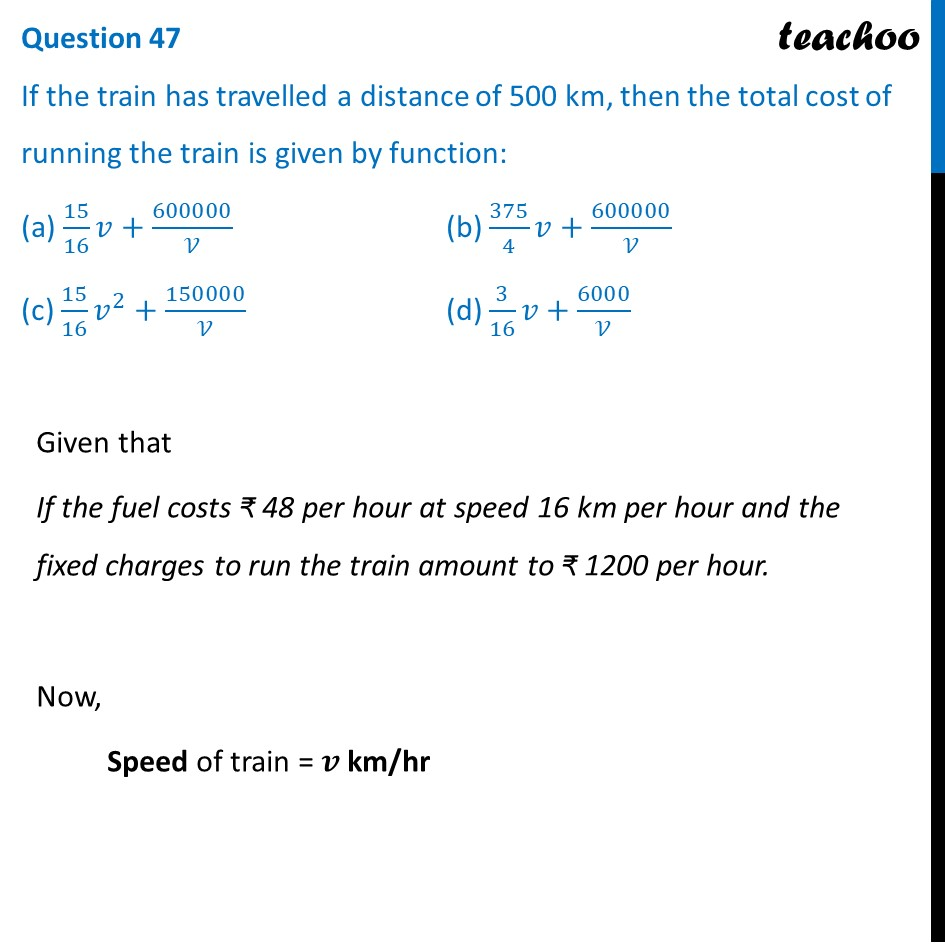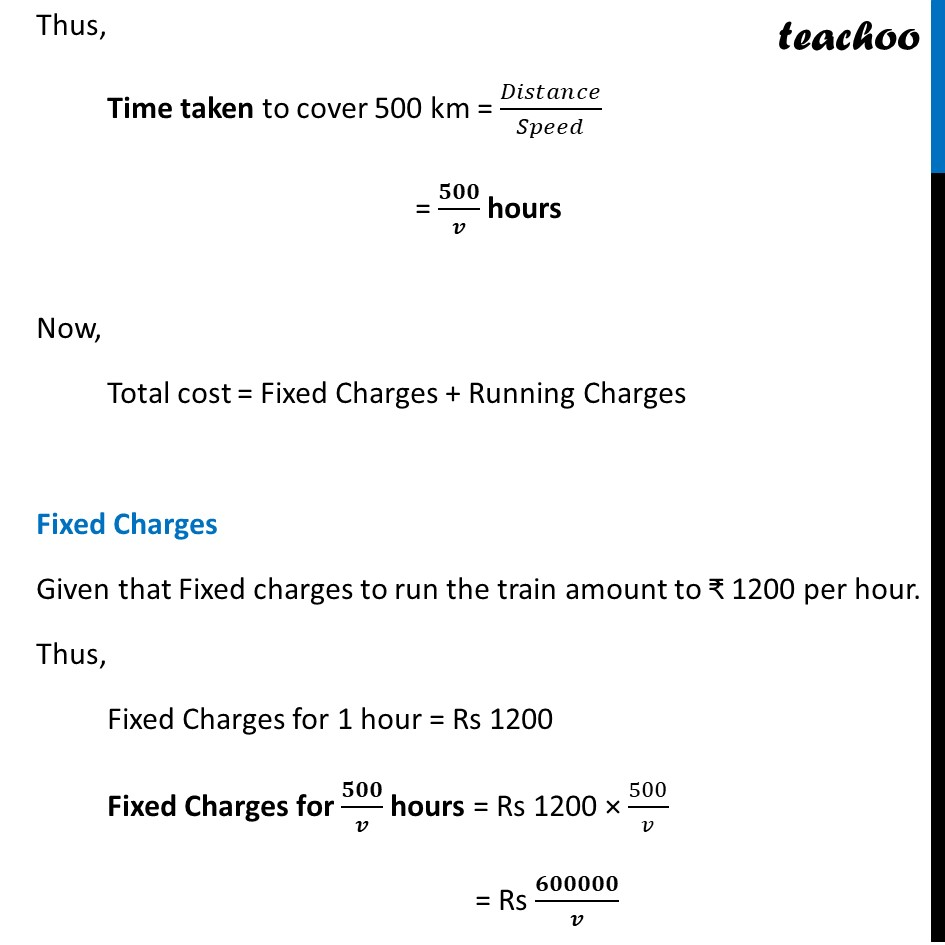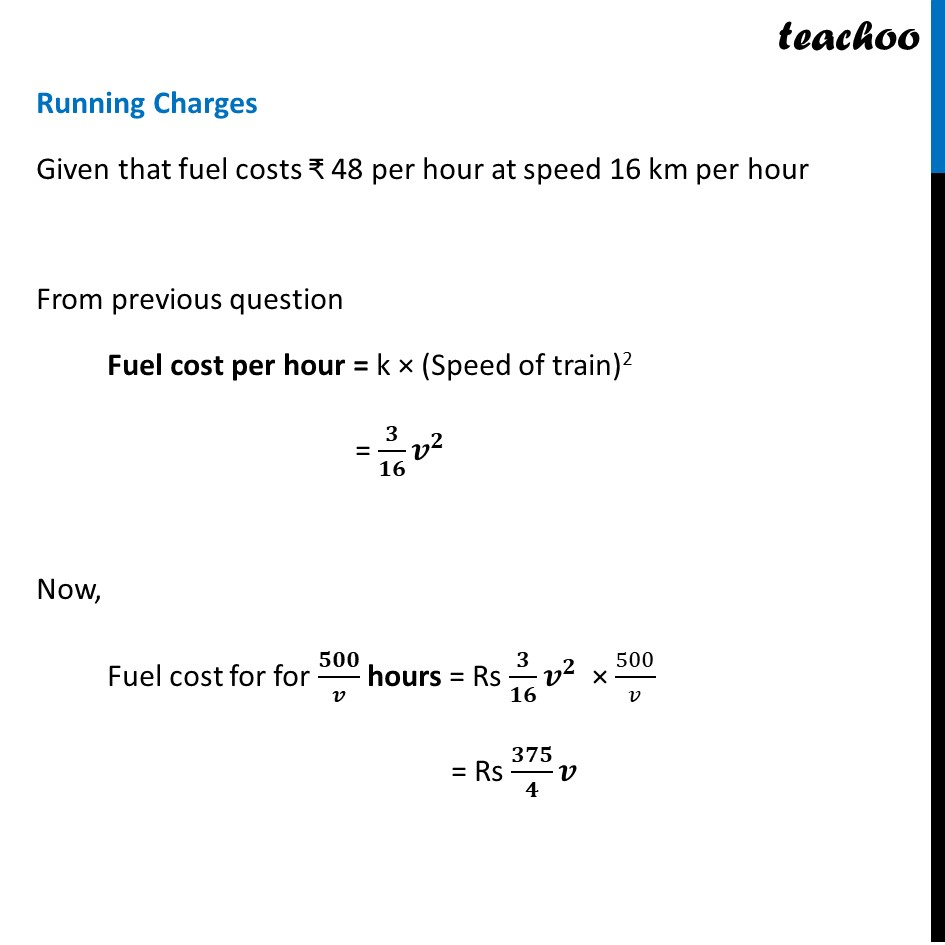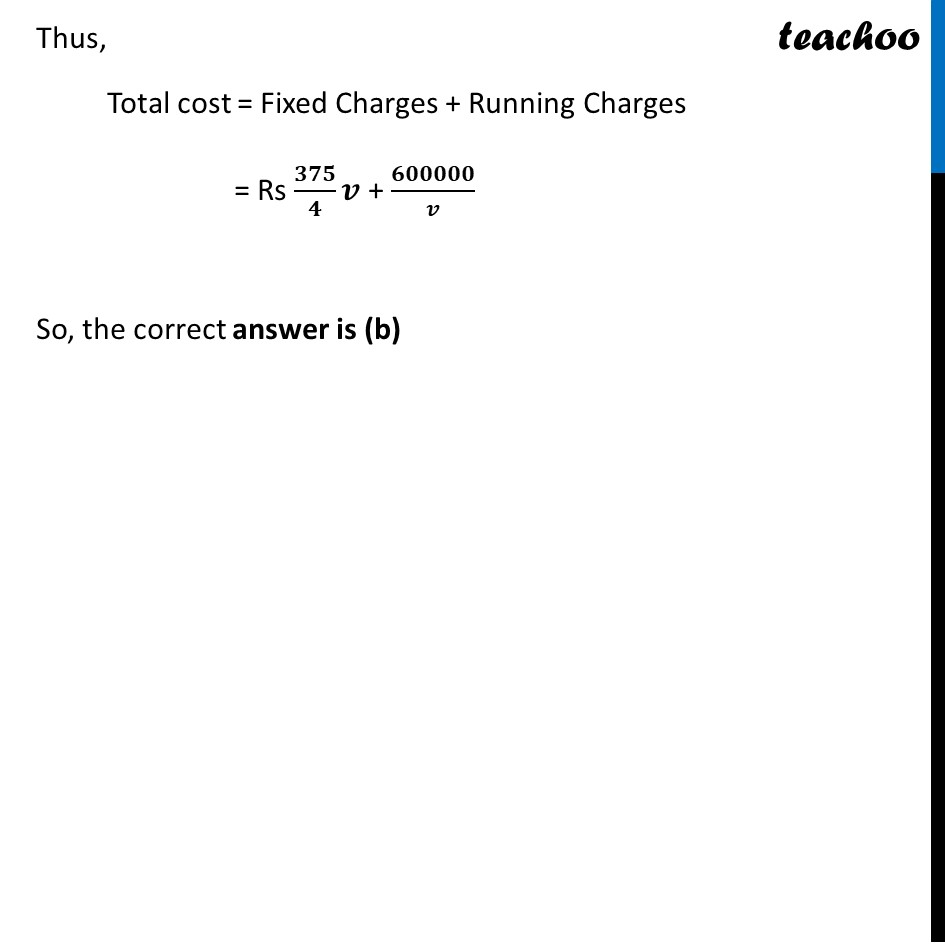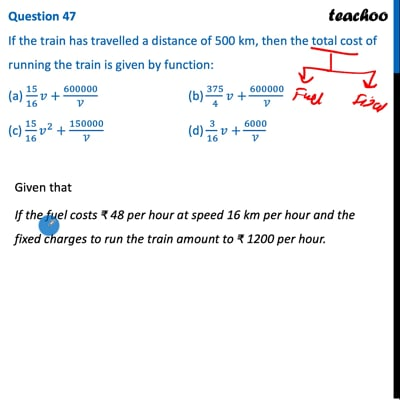This video is only available for Teachoo black users

Introducing your new favourite teacher - Teachoo Black, at only ₹83 per month

### Transcript

Question 47 If the train has travelled a distance of 500 km, then the total cost of running the train is given by function: (a) 15/16 𝑣+600000/𝒱 (b) 375/4 𝑣+600000/𝒱 (c) 15/16 𝑣^2+150000/𝒱 (d) 3/16 𝑣+6000/𝒱 Given that If the fuel costs ₹ 48 per hour at speed 16 km per hour and the fixed charges to run the train amount to ₹ 1200 per hour. Now, Speed of train = 𝒗 km/hr Thus, Time taken to cover 500 km = 𝐷𝑖𝑠𝑡𝑎𝑛𝑐𝑒/𝑆𝑝𝑒𝑒𝑑 = 𝟓𝟎𝟎/𝒗 hours Now, Total cost = Fixed Charges + Running Charges Fixed Charges Given that Fixed charges to run the train amount to ₹ 1200 per hour. Thus, Fixed Charges for 1 hour = Rs 1200 Fixed Charges for 𝟓𝟎𝟎/𝒗 hours = Rs 1200 × 500/𝑣 = Rs 𝟔𝟎𝟎𝟎𝟎𝟎/𝒗 Running Charges Given that fuel costs ₹ 48 per hour at speed 16 km per hour From previous question Fuel cost per hour = k × (Speed of train)2 = 𝟑/𝟏𝟔 𝒗^𝟐 Now, Fuel cost for for 𝟓𝟎𝟎/𝒗 hours = Rs 𝟑/𝟏𝟔 𝒗^𝟐 × 500/𝑣 = Rs 𝟑𝟕𝟓/𝟒 𝒗 Thus, Total cost = Fixed Charges + Running Charges = Rs 𝟑𝟕𝟓/𝟒 𝒗 + 𝟔𝟎𝟎𝟎𝟎𝟎/𝒗 So, the correct answer is (b)